# How to Determine the Eigenvectors of a Matrix

Instructor: Damien Howard

Damien has a master's degree in physics and has taught physics lab to college students.

In this lesson, you'll explore the subject of eigenvectors. After learning what an eigenvector is in concept, we'll solidify in your mind how to find them by working through an example problem together.

## Row Operations

As children we spent our time in elementary school math classes learning the various ins and outs of the mathematical operations: addition, subtraction, multiplication, and division. In linear algebra you learn these operations all over again. Only this time instead of working with scalars, you're working with vectors and matrices.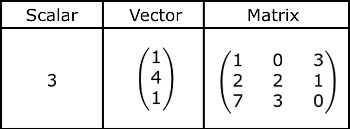If we look at a matrix and a scalar, we can see that the big difference between the two is that the scalar is made of one number and the matrix a bunch of rows and columns of numbers. In addition to learning how to use the four basic operations with matrices, there are an additional three operations that are used on the rows or columns within a single matrix. We call these the row operations, and they are as follows:

1) Any two rows within a matrix can be swapped.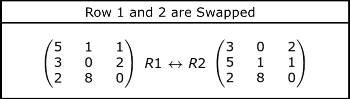2) You can multiply each entry in a row by a non-zero scalar.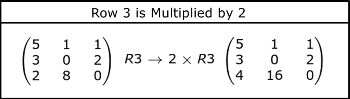3) You can add one row that's been multiplied by a scalar to another row.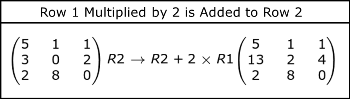Knowing how to do these row operations is extremely useful for working with matrices. One example of their usefulness comes into play when finding the eigenvectors of a matrix. In the rest of this lesson we'll learn what eigenvectors are, and how to find them for a given matrix.

## Eigenvalues and Eigenvectors

In order to understand eigenvectors, we also need to talk about eigenvalues. You cannot find a matrix's eigenvectors without first knowing its eigenvalues.

To see what eigenvalues and eigenvectors, are we start with the following fact. When you multiply a matrix (A) times a vector (v), you get another vector (y) as your answer.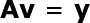Sometimes the vector you get as an answer is a scaled version of the initial vector.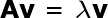When this happens, the scalar (lambda) is an eigenvalue of matrix A, and v is an eigenvector associated with lambda. We find the eigenvectors for a given eigenvalue by solving the following equation for v.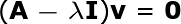In this equation, I is an identity matrix the same size as A, and 0 is the zero vector.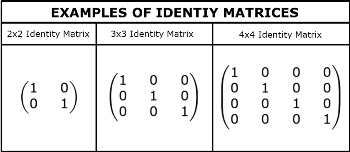To find all of a matrix's eigenvectors, you need solve this equation once for each individual eigenvalue.

## Example Problem

In order to see exactly how we solve for v, let's work through an example problem.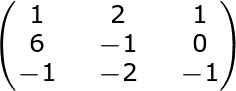The matrix above has eigenvalues (lambda) of 0, -4, and 3. We'll find the eigenvectors associated with lambda = -4.

To find this eigenvector, the first thing we need to do is insert this matrix and eigenvalue into our equation from the previous section and simplify the problem.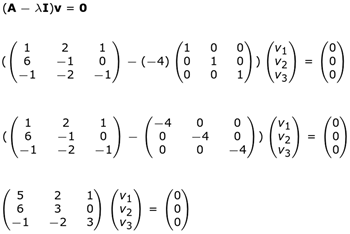The next step in the process is using Gaussian elimination to get our matrix in row echelon form. If you're not already familiar with those two terms, what I've just said probably sounded like gibberish. Let's break down exactly what we'll be doing.

First, what is row echelon form? A matrix is in row echelon form when the following three conditions are met:

1) All zero rows must be at the bottom of the matrix.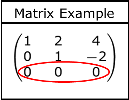2) The first entry of all non-zero rows is to the right of the first entry of the row above it.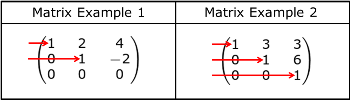3) The first entry of every non-zero row is a one.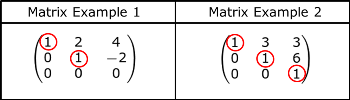In order to get a matrix in row echelon form, we use Gaussian elimination. This process has two steps. The first is to convert our equation into an augmented matrix.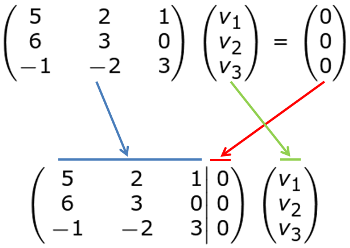The next step is where those row operations we went over at the beginning of this lesson finally see some use. We use the three row operations on our augmented matrix until we have it in row echelon form. When doing this yourself, the process may require some trial and error until you get the matrix in the right form.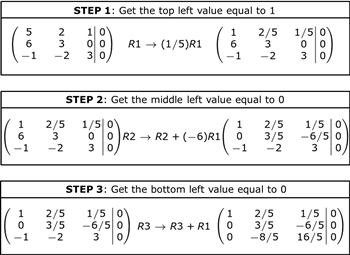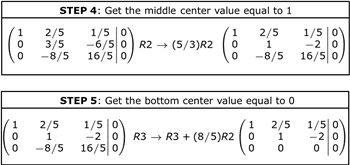To unlock this lesson you must be a Study.com Member.

### Register to view this lesson

Are you a student or a teacher?

### Unlock Your Education

#### See for yourself why 30 million people use Study.com

##### Become a Study.com member and start learning now.
Back
What teachers are saying about Study.com

### Earning College Credit

Did you know… We have over 160 college courses that prepare you to earn credit by exam that is accepted by over 1,500 colleges and universities. You can test out of the first two years of college and save thousands off your degree. Anyone can earn credit-by-exam regardless of age or education level.